# AlphaPose--Multip Personal Human Pose Estimation

This is my reading note for RMPE: Regional Multi-person Pose Estimation and the code is available at MVIG-SJTU/AlphaPose. This paper is a novel regional multi-person pose estimation (RMPE) framework to facilitate pose estimation in the presence of inaccurate human bounding boxes. The framework consists of three components: Symmetric Spatial Transformer Network (SSTN), Parametric Pose Non-Maximum-Suppression (NMS), and Pose-Guided Proposals Generator (PGPG).

Well, there is no place showing why it could be significantly faster than Mask-RCNN based approaches.

# Related Work

For human pose estimation methods, there are two types of algorithms:

• The two-step framework first detects human bounding boxes and then estimates the pose within each box independently. In the two-step framework, the accuracy of pose estimation highly depends on the quality of the detected bounding boxes.
• The part-based framework first detects body parts independently and then assembles the detected body parts to form multiple human poses. In the part-based framework, the assembled human poses are ambiguous when two or more persons are too close together. Also, part-based framework loses the capability to recognize body parts from a global pose view due to the mere utilization of second-order body parts dependence.

# Proposed Method

The propose method belongs to two-step framework, which is illustrated below: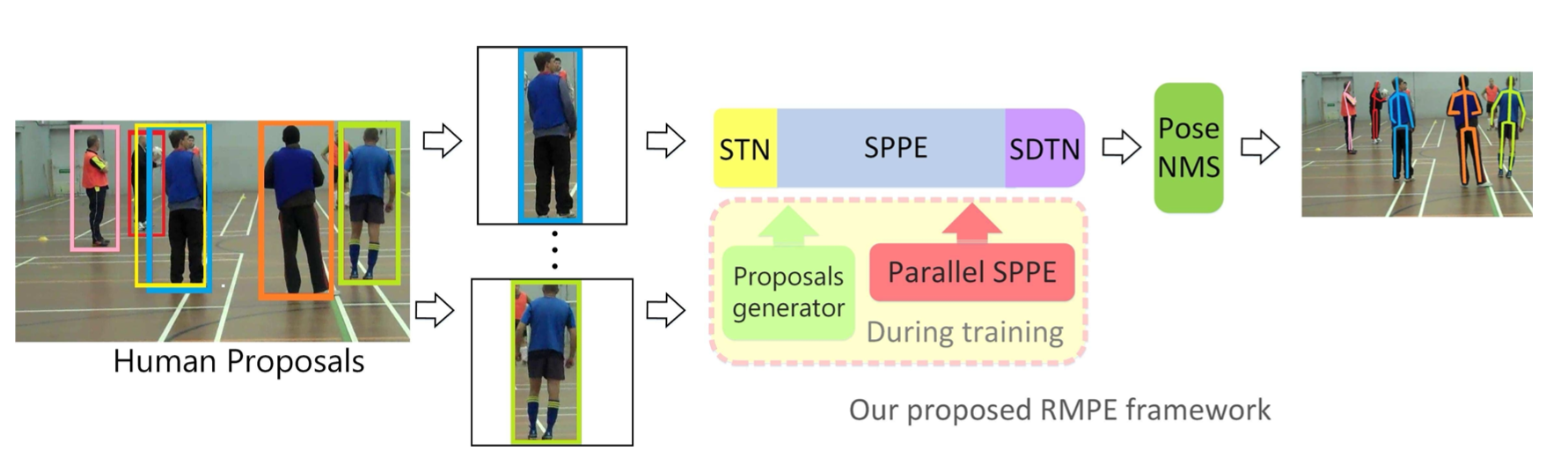## Symmetric Spatial Transformer Network (STN) and Parallel Single Person Pose Estimation (SPPE)

This paper uses the STN to extract high quality dominant human proposals. Mathematically, the STN performs a 2D affine transformation that transform the pose in image coordinate to pose in local bounding box. Naturally, a spatial de-transformer network (SDTN) is required to remap the estimated human pose back to the original image coordinate.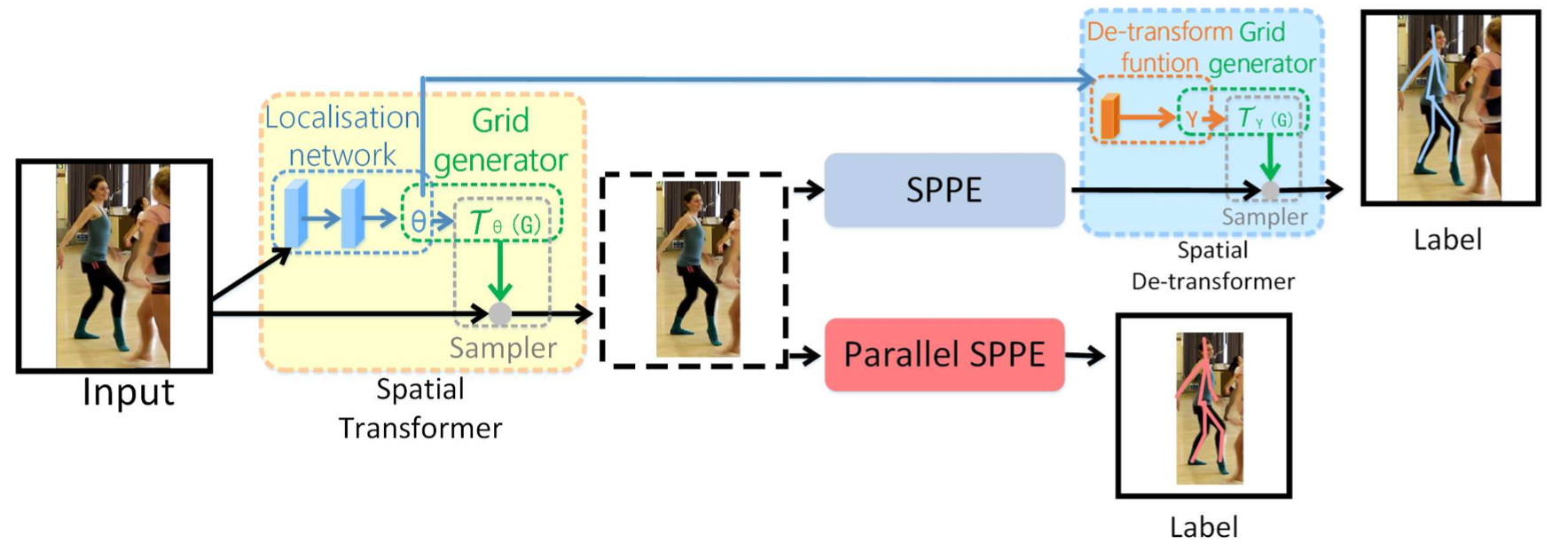### Parallel SPPE

The parallel SPPE can be regarded as a regularizer during the training phase. It helps to avoid a poor solution (local minimum) where the STN does not transform the pose to the center of extracted human regions. Although STN can partly transform the input, it is impossible to perfectly place the person at the same location as the label. The difference in coordinate space between the input and label of SPPE will largely impair its ability to learn pose estimation.

This branch shares the same STN with the original SPPE, but the spatial de-transformer (SDTN) is omitted. The output of this SPPE branch is directly compared to labels of center-located ground truth poses. We freeze all the layers of this parallel SPPE during the training phase. If the extracted pose of the STN is not center-located, the parallel branch will back-propagate large errors.

## Parameteric Pose Non Maximal Suppression (NMS)

NMS can be described as:

firstly, the most confident pose is selected as reference, and some poses close to it are subject to elimination by applying elimination criterion.

In this paper, the criterion includes both soft matching (similarity) function for pose (K) and spatial distance (H) between poses. The first function measures pose similarity and confidence; and the second function measures the spatial distance.

$K_{sim}(P_i,P_j|\sigma_1)=\left\{\begin{matrix} \sum_n{\tanh(\frac{c_i^n}{\sigma_1})\tanh(\frac{c_j^n}{\sigma_1})} & \mbox{if }k_j^n\in\mathbb{B}(k_i^n)\\ 0 & \mbox{otherwise} \end{matrix}\right.$ $H_{sim}(P_i,P_j|\sigma_2)=\sum_n{\exp[-\frac{(k_i^n-k_j^n)^2}{\sigma_2}]}$

Here $$P_i$$, $$P_j$$ are the poses, $$\mathbb{B}(k_i^n)$$ is the bounding box for key point $$k_i^n$$ (1/10 width of the bounding box for the person). Given the detected redundant poses, the four parameters in the eliminate criterion $$f(P_i,P_j\lvert\sigma_1,\sigma_2)$$ are optimized to achieve the maximal mAP for the validation set. This is why called parameteric NMS.

## Pose Guided Proposal Generator

The purpose is to generate large sample of training proposals with the same distribution as the output of the human detector. We find that the distribution of the relative offset between the detected bounding box and the ground truth bounding box varies across different poses.

However, considering the pose is high dimensional continous variable, directly modeling this distribution is impossible. As a result, atomic pose is used, which divided the whole pose space into several discrete poses. To derive the atomic poses from annotations of human poses, we first align all poses so that their torsos have the same length. Then we use the k-means algorithm to cluster our aligned poses, and the computed cluster centers form our atomic poses.

# Experiment Result

This is the ablation study for different components. Here we find PGPG is has the most impact to the final performance, followed by Pose NMS.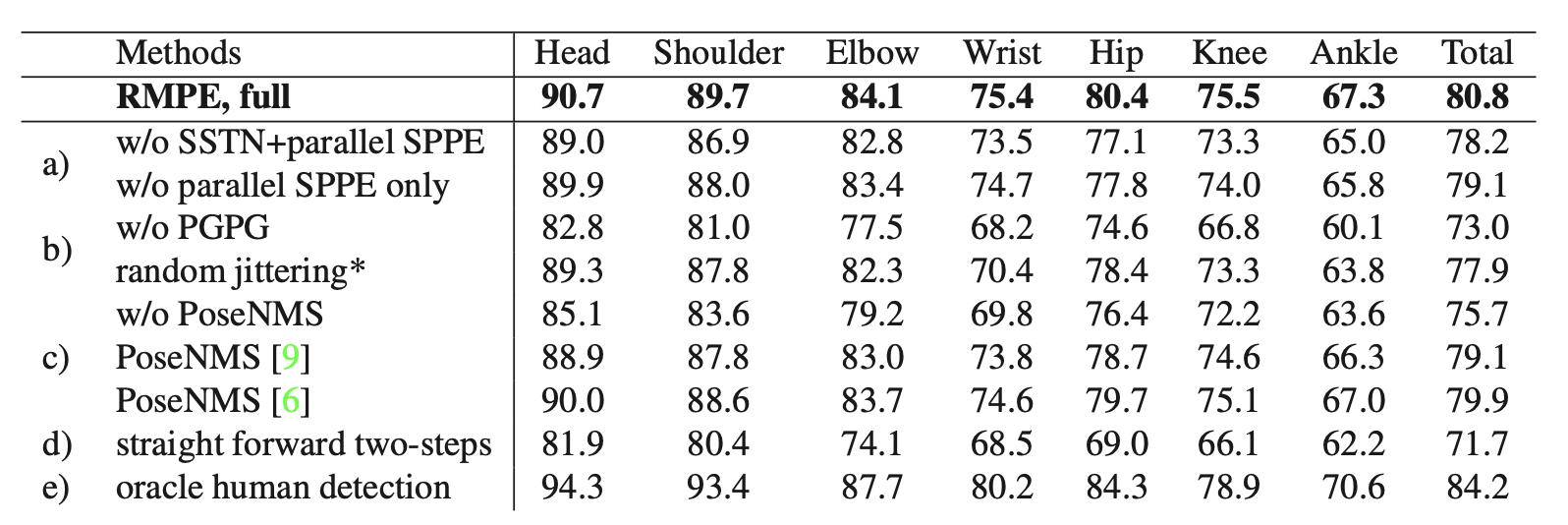This is result on coco.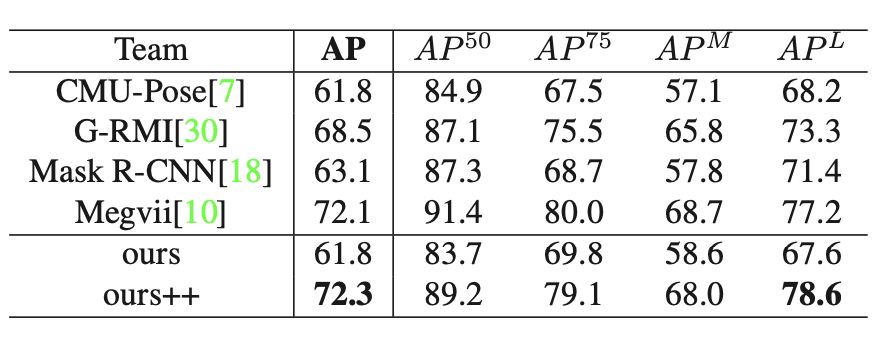Some visual examples: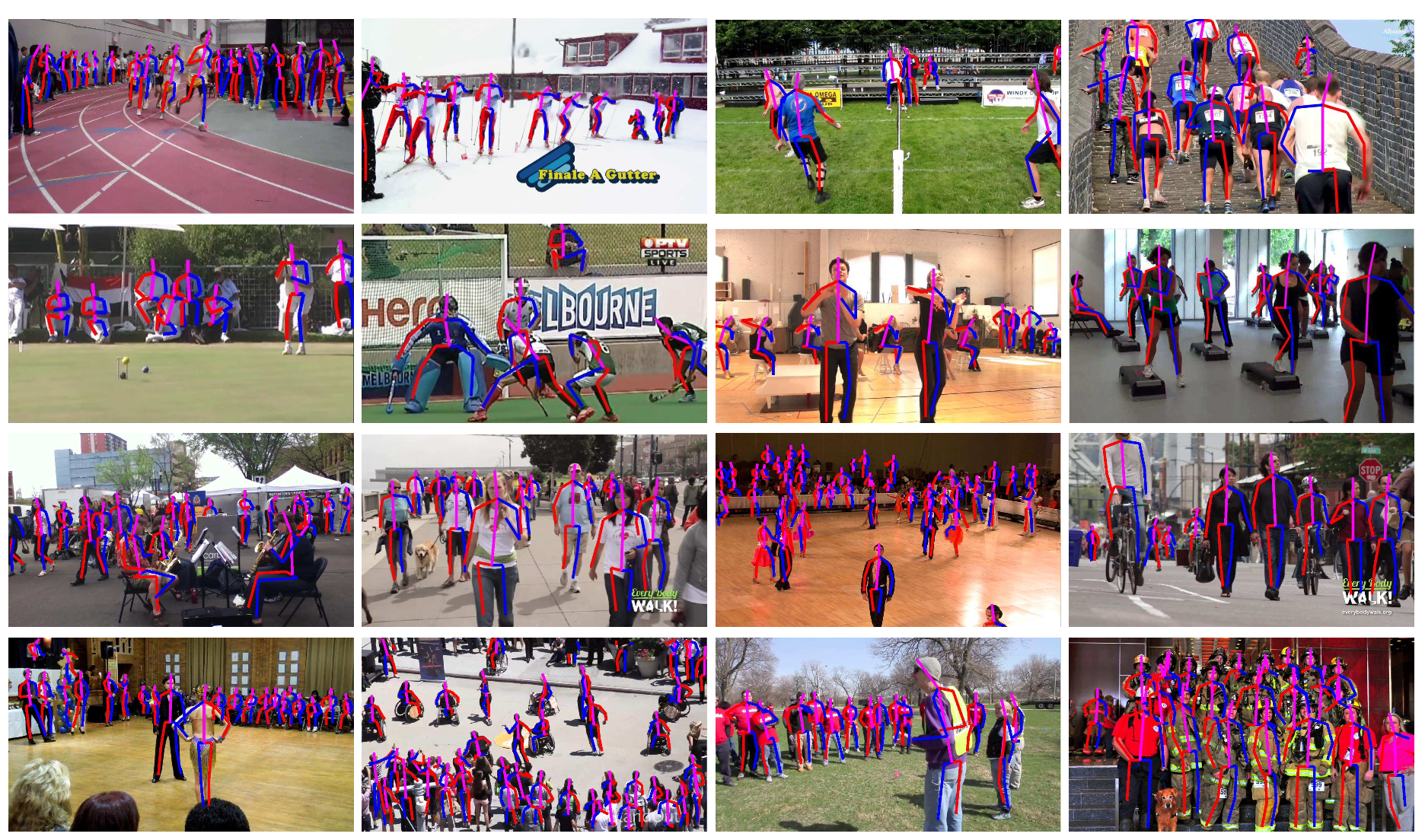Some failed cases: it can be seen that the SPPE can not handle poses which are rarely occurred (e.g. the person performing the ’Human Flag’ in the first image). When two persons are highly overlapped, our system get confused and can not separate them apart (e.g. the two persons in the left of the second image).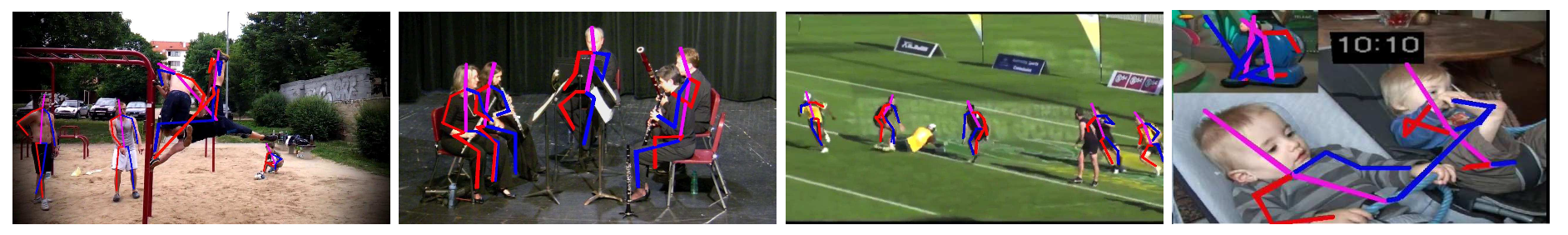Written on April 17, 2021